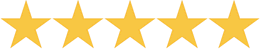A Beka Book Arithmetic 6 The teach/reteach approach and abundant practice problems in this work-text help your student to master skills and concepts. Story problems and frequent problem solving strategies make arithmetic practical. Some major concepts are fractions, decimals, proportions, percents, prime factoring, algebraic equations, measurement, basic geometry, and beginning banking. Soft cover.
 Can You Count in Greek? Judy Leimbach This thorough introduction to ten different ancient number systems is a valuable resource for math enrichment or an introduction to our number system or incorporate it into any Ancient history unit.Price thisChallenge Math A unique new resource for children gifted in math. Your child will love the fascinating true stories that tie math and science together and will be challenged by over 1000 problems at three levels of difficulty. Parents will appreciate the easy to learn instructional format that makes teaching and learning enjoyable. Contains 350 pages and twenty chapters in astronomy, statistics, probability, algebra, physics, and more. Allows children to see the wondrous side of math and its important role in today's world. A great way to enrich your curriculum.Price thisKey to Algebra Books Julie King Key to Workbooks offer a positive alternative to large, expensive textbooks which students often find complicated and intimidating. Only one concept is presented per page, so students have time to understand new ideas without being overwhelmed. Hand-written examples and exercises make work seem less formal. Vocabulary and reading levels are simple. Instructions are clear and direct. Each book is a complete text-workbook. Students develop understanding by solving equations & inequalities intuitively before formal solutions are introduced. a Full year's course that stands entirely on its own.(5)
1 ReviewPrice thisBob Jones University Press Math 6 Develops further understanding of multiplication and division of fraction concepts, proportions, percents, and statistics. Includes a pre-algebra chapter, which introduces multiplication and division of integers using manipulatives. Continues to emphasize problem-solving and estimation skills. Teacher's Edition contains 165 lesson plans divided into 18 chapters. Includes skill practice activities, a spiraling review, and teaching examples. Provides additional information about memorizing facts, bulletin board ideas, and mental math computations. Teacher's Edition: 966 pages; two spiral binders. Student Text features activities using the theme ''Courage in Crisis'' with Jay Janson, a sixth grader, and his family. Student Text: 416 pages; hardbound; full color. Student Materials Packet provides colorful manipulatives to be used in the lessons: two-color counters, fraction kit, Base Ten kit, a number line with positive and negative numbers, and more.(5)
1 Review

 Saxon Math 7/6 Excellent K-12 textbook and workbook math program. Students will generally work independently. Uses an effective incremental learning model.Price thisMath By Kids Written by Pennsylvania Homeschoolers. This unusual book of word problems was written by homeschool students of all ages. Arranged by categories - Math Problems About Food, Working and Earning, Mystery Numbers, Etc. Problems are listed from easiest to hardiest.Price thisMath Drillsters Bob Bernstein A reproducible activity book containing 220 pages of math drill activities primarily covering addition, subtraction, multiplication, division, but also touching on measurement, time, money, temperature, fractions and mathematical thinking.